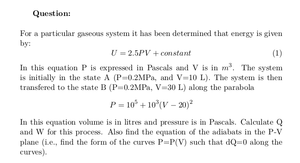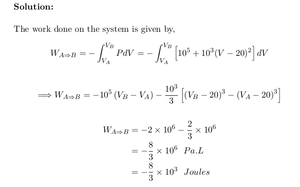Solved Problems in Physics, Physical Chemistry, and Computer Simulation Projects for College and Graduate Students.# Transition of a Gaseous System Through a Parabola

Regular price
\$1.99
Sale price
\$1.99
Tax included.

Question:

For a particular gaseous system it has been determined that energy is given by:   U = 2.5P V + constant

In this equation P is expressed in Pascals and V is in m3. The system is initially in the state A (P=0.2MPa, and V=10 L). The system is then transfered to the state B (P=0.2MPa, V=30 L) along the parabola  P = 105 + 103 (V − 20)2 .

In this equation volume is in litres and pressure is in Pascals. Calculate Q and W for this process. Also find the equation of the adiabats in the P-V plane (i.e., find the form of the curves P=P(V) such that dQ=0 along the curves).

By purchasing this product, you will get the step by step solution of the above problem in pdf format and the corresponding latex file where you can edit the solution. I am also available to help  you with any possible question you may have.